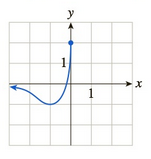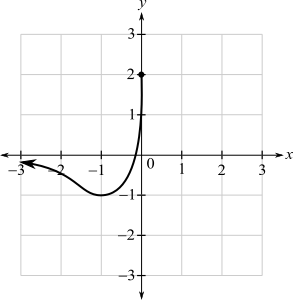Chapter 10.1, Problem 44EFinite Mathematics and Applied Cal...

7th Edition
Stefan Waner + 1 other
ISBN: 9781337274203

Solutions

Chapter
SectionFinite Mathematics and Applied Cal...

7th Edition
Stefan Waner + 1 other
ISBN: 9781337274203
Textbook Problem

In Exercises 35–48 the graph of f is given. Use the graph to compute the quantities asked for. [HINT: See Examples 4–5.]a. lim x → − 1 f ( x ) b. lim x → o − f ( x ) c. lim x → 0 f ( x ) d. f ( 0 )(a)

To determine

The value of limx0+f(x) by the use of the graph which is as follows:Explanation

Given information:

The graph is as follows:

From the graph, to evaluate the limit for x tends to 0+ for the function f(x)

(b)

To determine

The value of limx0f(x) by the use of the graph which is as follows:(c)

To determine

The value of limx0f(x) by the use of the graph which is as follows:(d)

To determine

The value of f(0) by the use of the graph which is as follows:Still sussing out bartleby?

Check out a sample textbook solution.

See a sample solution

The Solution to Your Study Problems

Bartleby provides explanations to thousands of textbook problems written by our experts, many with advanced degrees!

Get Started

Show that the equation has exactly one real root. x3 + ex = 0

Single Variable Calculus: Early Transcendentals, Volume I

Prove that limx15(x+1)3=.

Single Variable Calculus

The polar form for the graph at the right is:

Study Guide for Stewart's Single Variable Calculus: Early Transcendentals, 8th